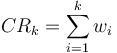# Concentration Ratio

## Definition

For the purpose of measuring credit portfolio or market Concentration Risk, diversity or inequality metrics the concentration ratio is a measure of the contribution of a given set of exposures to the total portfolio exposure - essentially the portfolio fraction. For example the fraction of exposure contributed by the top ten largest clients, the contribution to the market capitalization by the top five ten largest listed companies etc.

## Details

The concentration ratio is simply the percentage of portfolio exposure by the n largest exposures. Assuming the exposure data are given, calculation is easy.

The ratio associated with the contribution of k entities (in a portfolio or sample of size n > k) is denoted as$CR_{k} = \sum^{k}_{i=1} w_{i}$

where wi is simply the share of the i-th exposure in the total portfolio exposure.

### Berger Parker Index (special case)

In diversity studies, the special case k=1 is denoted as the Berger-Parker index. It is the fraction of the population formed by the most abundant category.

## Usage

Along with the Herfindahl-Hirschman Index (HHI) the concentration ratio is a tool typically used by competition authorities to measure market concentration.

These tools are useful also in the context of analysing portfolio concentrations, for example credit or market risk concentrations.

The concentration ratio is a "relative" measure, i.e., all exposures are normalized as fractions of total portfolio exposure, therefore two portfolios with same index are deemed to be equally concentrated irrespective of their absolute total size.

### Common Ratios

There is no intrinsic rule to select the number n of exposures to assess. Some commonly used examples include

• The n=1 Concentration Ratio measures the total contribution of the largest exposure (equivalent to the Berger-Parker index).
• The n=4 Concentration Ratio measures the total contribution of the four largest exposures.
• The n=8 Concentration Ratio measures the total contribution of the eight largest exposures.
• The n=20 Concentration Ratio measures the total contribution of the twenty largest exposures.
• The n=50 Concentration Ratio measures the total contribution of the fifty largest exposures.

The case n=20 is important for the purposes of credit risk concentration analysis because it is used in the Large Exposures Framework

### Interpretation and Concentration levels

Concentration ratios can range in value from 0 to 100 percent.

Whether a given result indicates a concentrated portfolio cannot be assessed beforehand but only in relation with typical portfolios, standard market practices etc. or other metrics. For example the Large Exposures Framework links the size of the top 20 large exposures to the capital of the firm.

Concentration levels can characterized as no, low or medium to high to "total" concentration based on subjective thresholds.

### Advantages

Key advantages of the concentration ratio index include

• Its conceptual and computational simplicity. Indeed even a casual glance at the sorted and normalized (divided by total) exposures provides already an intuitive feeling as to the degree of concentration in a given portfolio and
• The relatively moderate data requirements, i.e. only the exposure size per borrower (but see qualification below)

### Disadvantages

• The definition of the concentration ratio must pick a set of representative entities (the number n) to assess concentration and does not use the contribution of all exposures. A portfolio may look acceptable for a given n, but less so for another choice.
• The index suffers from a one-dimensional view of concentration risk, namely it is assumed that relative size is the only factor worthy of consideration. In reality there may be a wide range of other factors
1. Exposure size may not be obvious to define
2. Risks associated with different exposures can be very different
3. There may be varying degrees of dependency among different exposures
• It is difficult to objectively characterize the concentration thresholds.

The Herfindahl-Hirschman Index and other indexes provide more complete picture of concentration than does the concentration ratio, thereby mitigating the first of the above disadvantages

None

## Issues and Challenges

Although the data requirements for the concentration ratio calculation seem to be moderate at first sight, they often turn out to be difficult to meet in practice. They require the aggregation of all exposures to the same borrower for the whole business entity, be it a bank or a banking group. A heterogeneous IT environment can already present a serious technical obstacle to this aggregation. Furthermore, large borrowers, which are actually the most relevant entities for measuring name concentration, can themselves be complex structures that are not always easy to identify as belonging to the same borrower entity.

## Implementations

Open Source implementations of the Concentration Ratio are available in

None